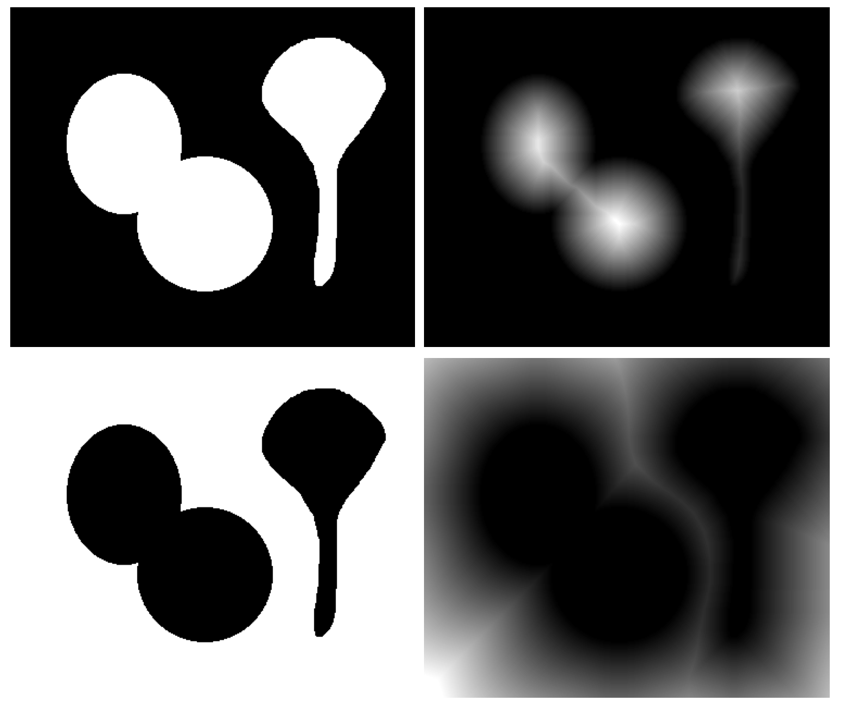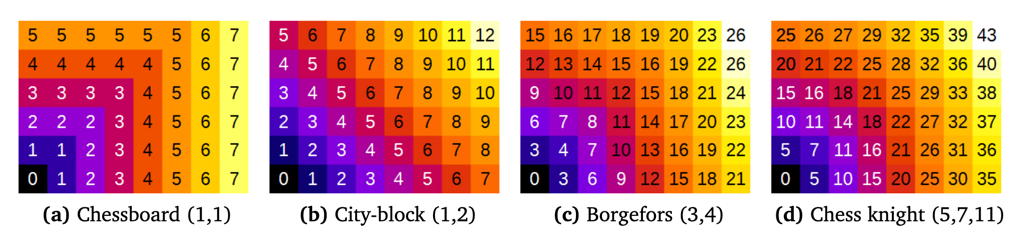## Prerequisites

Before starting this lesson, you should be familiar with:

## Learning Objectives

After completing this lesson, learners should be able to:
• Understand how to use distance transform to quantify morphology of objects

• Understand how to use distance transform to quantify distance between objects

• Understand approximate nature of distance transform

## Motivation

We use distance transform to quantify how a structure of interest is away from object boundaries or other structures. The distance transform is also use to characterize the morphology of an object in 2D and 3D, find its center, dimensions, etc.. Finally distance transform can be used as a pre-processing step to improve the segmentation results and split touching objects.

## Concept map

graph TD B[Binary image] --> DT(Distance transform) DT --> D["Distance map image"] D -- contains --- DN("Distances to nearest background pixel") D -- is --- A("Approximation of euclidian distance") DT -- has --- VI("Various implementations")

## FigureUpper panel - Binary image and the corresponding distance map. The distance map has three local maxima, which are very useful for object splitting and defining object centers. Lower panel - Inverted binary image and corresponding distance map, which is useful to compute distances to closest objects.

## Activities

### Distance transform basics

• Perform a distance transform.
• Invert the binary input image.
• Perform another distance transform on the new binary image.
• Observe that the datatype (in particular 8-bit) of the distance transform image limits the distances.
• Discuss different metrics.
• Add a calibration to the inverted image and recompute the distance transform.
• Observe whether the image calibration is considered for the distances.

### Distance measurements

• Create an inverted binary mask for one of the labels (e.g. label 1)
• Compute the distance transform
• Measure intensity (= distances to nearest other objects) of objects in the distance map
• Show that the measured values correspond to the distance of label 2 and 3 to label 1.

### Region selection by distance gating

• Open reference object image: xy_8bit_binary__single_object.tif.
• Invert the binary
• Compute the distance transform
• Perform an intensity gating on the distance map, e.g. by thresholding, to create a mask with all pixels of a certain distance, e.g. between 100-120, to the reference object.

Show activity for:

## Basics, ImageJ GUI MorpholibJ

### Distance map using default ImageJ distance transform

• [Image > Duplicate…]
• `Title: "Distance Map"`
• [Process › Binary › Distance Map], note that the resulting image is 8-bit

• Select the `binary` window
• [Image > Duplicate…]
• `Title: "inverted"`
• [Edit > Invert]
• [Image > Duplicate…]
• `Title: "Distance Map inverted"`
• [Process › Binary › Distance Map], discuss the results and how far away pixels have always a value 255!

### Distance map using MorphoLibJ

• Select window `inverted`
• [Plugins › MorphoLibJ › Binary Images › Chamfer Distance Map]
• Discuss the menu and the fact that there are different options for the metric.
• Preview the different distances and how you get unexpected values for certain distances
• Run the distance transform with following options
• `Distances: Borgefors (3,4)`
• `Output Type: 16 bits`
• `[x] Normalize weights`
• [Image > Rename]
• “Borgefors”
• [Plugins › MorphoLibJ › Binary Images › Chamfer Distance Map]
• Discuss the menu and the fact that there are different options for the metric.
• Preview the different distances and how you get unexpected values for certain distances
• Run the distance transform with following options
• `Distances: Chessknight (5,7,11)`
• `Output Type: 16 bits`
• `[x] Normalize weights`
• [Image > Rename]
• “Chessknight”
• Discuss the obtained values

## Exercises

### Measure distance to center of cell

We would like to measure the distance within a binary mask to a specific point in the cell. This is called Geodesic distance.

### Measure thickness of glial branches

The goal is to combine skeletonization and distance map computation to measure skeleton branch length and thickness. For this exercise you need the binary image xy_8bit_binary__glialcell.tif and the skeletonized and normalized version xy_8bit_binary__glialcell_skeleton.tif

• Compute the distance transform of the binary
• Multiply the skeleton image with the distance map
• Perform an analyze of the skeleton
Show exercise/solution for:

## Distance to center, ImageJ GUI

• [File > Open…] xy_8bit_binary__glialcell.tif
• You need to define a reference marker using the point ROI (in the Fiji menu 7th element from the left). Place it close to the center of the cell.
• [Plugins › MorphoLibJ › Binary Images › Interactive Geodesic Distance Map]
• `Distances: Chessknight (5,7,11)`
• `Output Type: 32 bits`
• `[x] Normalize weights`
• The image values correspond to the distance to the reference point within the binary mask.

## Glial thickness, ImageJ GUI

• [File > Open…] xy_8bit_binary__glialcell.tif
• Compute the distance map using the default plugin (we need a 8 bit image for the analyze skeleton step)
• [Process > Binary > Distance Map]
• [ Image › Rename…]
• “Distance Map”
• [File > Open…] xy_8bit_binary__glialcell_skeleton.tif
• [ Image › Rename…]
• “Skeleton”
• Use the image calculator function [ Process › Image Calculator…] to multiply the skeleton image by the distance map:
• Image1: Skeleton
• Operation: Multiply
• Image2: Distance map
• ‘create new window’
• ‘32-bit float result’
• [ Image › Lookup Tables › Fire]
• Obtain branch information by analyzing the skeleton: [Analyze › Skeleton › Analyze Skeleton (2D/3D)]
• ‘Prune ends’
• ‘Calculate largest shortest path’
• ‘Show detailed info’
• ‘Display labeled skeletons’.
• In the ‘Branch information’ table, you can find information on branch length, as well as average intensity. Since the distance map tells you the distance a pixel is away from the boundary, you can estimate the average branch thickness by multiplying this value by 2.

## Assessment

1. Knowing the image calibration, how could we convert a 2D distance map to physical values?
2. Knowing the image calibration, how could we convert a 3D distance map to physical values? Is it important if the image is isotropic sampled or not?

## Explanations

#### Chamfer distance (modified from MorpholibJ Manual)Several methods (metrics) exist for computing distance maps. The MorphoLibJ library implements distance transforms based on chamfer distances. Chamfer distances approximate Euclidean distances with integer weights, and are simpler to compute than exact Euclidean distance (Borgefors, 1984, 1986). As chamfer weights are only an approximation of the real Euclidean distance, some differences are expected compared to the actual Euclidean distance map.

Several choices for chamfer weights are illustrated in above Figure (showing the not normalized distance).

• The “Chessboard” distance, also named Chebyshev distance, gives the number of moves that an imaginary Chess-king needs to reach a specific pixel. A king can move one pixel in each direction.
• The “City-block” distance, also named Manhattan metric, weights diagonal moves differently.
• The “Borgefors” weights were claimed to be best approximation when considering the 3-by-3 neighborhood.
• The “Chess knight” distance also takes into account the pixels located at a distance from (±1, ±2) in any direction. It is usually the best choice, as it considers a larger neighborhood.

To remove the scaling effect due to weights > 1, it is necessary to perform a normalization step. In MorphoLibJ this is performed by dividing the resulting image by the first weight (option `Normalize weights`).

## Follow-up material

Recommended follow-up modules: